## Sunday, 27 November 2011

### Making a sacrificial bid

IMP pair, nil vul, N opening.
North
S ---
H 96
D 84
C AQJT98762

South
S AJ9852
H AKQ72
D -----
C K4

A clear 7C you would say, despite that my (robot) opponent is stupid and didn't make any signal for his long solid C. A suitable calling should be like this
1C - X - 1H - /
3C - 3D - 3S - 5D
/ - / - X - /
7C A.P.
 - solid C (solid 6+) or 13P+, 3+C
 - t/o double
 - very solid C (7.5 winner) and weak hand
 - 5-4 or above good major suit, 16+, GF
 - D support
 - DOPI, pass = 1 A (probably C)
 - D first control, decide between 5DX, 6C or 7C
OR like this if you like the gambaling conventions
3NT - / - 4H - /
5C - / - 7C A.P.

 - Gambaling 3NT, solid minor with no side power
 - promising power, 5+ strong H, slam interest
 - the suit is C
 - To play

...and the stupid robot play like this
/ - 1D - X - /
2C - 2D - 4D - 5D
6C  A.P.

 - unknown response by bot
 - t/o double
 - 4+C
 - variation of splinter
 - without detailed discussion

What makes 7C biddable is that:
- A long, solid C with 9 winner including C K
- Voiding D
- A fine 5-5+ major with first control

So which one you would prefer? Difficulties are always experienced when you hold a long C if you use natrual/SAYC system because 2C is artificial strong 2C but not weak 2.
Instead, we give 1C another artificial meaning [to show long C] followed by recall signal. The resulting C is better than usual weak 2, or even exceeding 3X weak call.
On another side, South has to show his fine distribution and support. A 1H-3S is used in the first bidding approach, but for the second and third approach, we can only show "promising power" without detail of the hand due to the lack of biddable space.

For me, I prefer the second one since the 3NT suscessfully blocks almost all preemeptive bidding from the opponents as they failed to told their partner that they have a long D suit.

In reality, East holds:
S KQT
H JT4
D AKQ76
C 53
With opening power, his is willing to call out his diamond suit, and W is able to sacrifice to 7D (which is ~ -1100, less than 7C= which is ~-1400) with 11 D and some side power. E-W can reach 7DX-5.

Since West is the fourth bidder, it's not so possible for him to call out his D suit (and he's not even able to bid weal 2 2D opening). It relies heavily on how East showing his D hand. On the first and third approach the opponents reached 5D, but none of them reached 7D because E lacks confident.

In fact, whe you hold East's hand, knowing that North have a long solid C while South have a bunch of winner on major with first control, and South voids D (because he have first control but NOT D A), then West would have no power other than JXXXXX diamonds, by the law of total tricks, it's certain that 7D would work.

In reality, 6C+1 is made, 2 table reached 7C=, 1 table 7HX= (I'm surprised by this one), 1 table 7DX-5, 5 table 6C= with some table playing other contract and lose quite a lot of IMPs.

## Saturday, 19 November 2011

### NSS Mathematics: Co-geom techniques

﻿
This is a question from the latest test. A, B H are points (-6,0), (4,0), (0,10) respectively. C1 and C2 are circles with AO and BO as diameter respectively, AH and BH meet C1 and C2 at F and G respectively.
ai) Show OGHF concyclic.
ii) Show AFGB concyclic.
bi) Find coordinate of G.
ii) Find circle containing AFGB.

We will focus on part b as it includes all method that we can solve a problem related to circle in the co-geom plane.

bi) Find coordinate of G.

Method 1: Circle-line intersection
Recall the equation of C2: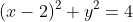Equation of line BH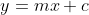, putting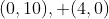we get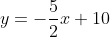.
Putting equation of line BH into equation C2: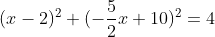.
By simplification we have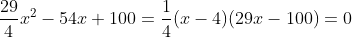. (4,0) is B so x=4 is rejected. Then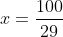, and we can easily get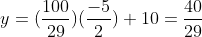.

Method 2: (the easiest one) perpendicular line interesection
Observe that OG is perpendicular to BH due to angles in semi-circle,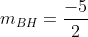,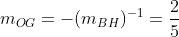. Since OG passes through the origin,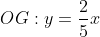.
The intersection between line BH and line OG: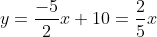, we can easily obtain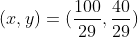.

Method 3: perpendicular line-circle intersection
The intersection between OG and C2 might me a bit easier than intersection between BH and C2: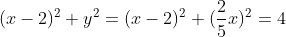, x = 0 (rej. since it's O) and the same result as before.

Method 4: trigonmetry method
Observe the triangles HOB, HGO and OBG are similar. Let angle GOB = angle HOB be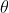and the coordinate of G is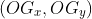.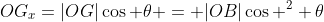, similarly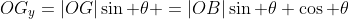.
By definition we have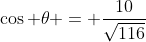,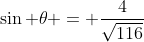, by putting |OB| = 4 we have the same result.

(Note: |XY| is the length of line segment XY.)

bii) Find the equation of circle:

Method 1: general equation of circle
Assume the equation is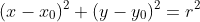.
Putting point A and B we have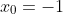, therefore the equation becomes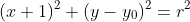and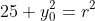.
Putting, we have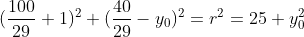After a bunch of complex calculation (ugly number), we have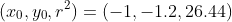, therefore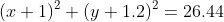is the desired equation.

Method 2: Perpendicular bisector method
Recall the way you determine the circumcenter: it's the intersection point among three perpendicular bisectors. Therefore if four points are concyclic, choose two perpendicular bisector of them, then insection point is the center of circle. The radius can be easily determined by distance formula.

The perpendicular bisector of AB is trivially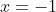.
The perpendicular bisector of BG is given by the locus of P that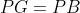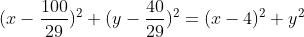By putting x = -1, we have y = -6/5, and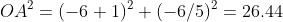, and the same result is given.

Conclusion:
1) Change circle problems into linear problems whenever possible.
2) Trigonometry is powerful when there's perpendicular pair of lines.
3) Finding intersection between circles is stupid.
4) Find the equation of circle in terms of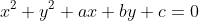is stupid.
5) Perpendicular bisector is our new method to find equation of circle.

fin.

## Sunday, 13 November 2011

### Chemistry notes: Industrial process

To access the document version of this chapter of notes click the "Notes Corner" above.

Industrial process
1)       Ammonia (nitrogenous plant)
Nitrogen acts as an important role in plants like producing amino acid. N2 in air can't be directly absorbed by plants while ammonia or nitrate has to be used. Artificial fertilizers are necessary to support intensive farming. The two fertilizers straight N (only nitrogen compounds are useful) and NPK (nitrogen, phosphorus and potassium containing in different ratio) can be used in different crops. Common fertilizers include NH4NO3, (NH4)2SO4, NH4PO4, H2NCONH2 (urea). Different solubility gives them different application in planting different crops.
P content is prepared from NH3 + H3PO4, while phosphoric acid can be produced from raw phosphorus and sulphuric acid. K content is prepared from KCl.
Nitrogen fixation process (which converts N2 in air into useful chemicals)
-          Drastic environment for reaction to occur, like N2 + O2 2NO in lightening and car engine
-          Action of bacteria: including nitrifying bacteria in root nodules converting nitrogen in atmosphere into nitrate ions
-          Haber process
Preparation of H2 and N2:
N2: by fractional distillation from air.
H2: by reduction of water by methane: CH4 + H2O 3H2 + CO, CO + H2O H2 + CO2, note that the proportion between methane and water must be controlled carefully to prevent production of side products. Also, water steam is used, NOT in liquid form.
Reaction flow:
I)                     Purifying and drying (remove CO, CO2 from poisoning catalyst), then compress the gas
II)                   Pump into heat exchanger and absorb heat and react in catalytic convertor
III)                  Haber process is exothermic, so excess heat is stored in heat converter
IV)                Ammonia is condense and stored, unused H2, N2 is pumped back into heat exchanger
Condition: 400~450 degree Celsius, 200 atm with finely powdered Fe catalyst.
Cost of process is reduced by recycling unused reactant and use hot product to warm up reactants.
Nitric acid HNO3 is prepared by NH3 by:
I)                     4NH3(g) + 5O2(g) 4NO(g) + 6H2O(g) in 900 degree Celsius, 10atm and Pt-Rh catalyst. In lab we can blow O2 into conc. NH3 with red-hot Pt-Rh catalyst hung in the beaker. The exothermic reaction gives enough heat for the catalyst to maintain high temperature. Beware that the reaction is explosive (enthalpy extremely negative)
II)                   2NO + O2 2NO2 N2O4 in 40 degree Celsius (or r.t.), 7-12 atm.
III)                  3N2O4(g) + 2H2O(l) 4HNO3(aq) + 2NO(g), note that cold water is used, while NO can be reused.
Industrial consideration
-          Storage: cost of tanks (chemical reactivity of chemicals like flammability, temperature and pressure needed), land cost, import cost, etc.
-          Transportation: cost of pipes (pressure, temperature, continuous VS patch)
-          Effect of heat on rate of reaction; to process purification, to dry the chemicals, to process water treatment
-          Separation and process control
Environmental problem from excess usage of fertilizers:
-          Nitrates are very soluble in water. Through underground water, water with high nitrate content went into water streams and river, causing eutrophication where algae disastrously grow, which releases toxics, uses up oxygen in water and destroy the whole environment.
-          When human drinks water with high nitrate contents, nitrate bond with hemoglobin and causing suffocation especially in baby. Since the bonded hemoglobin is blue, it's called blue baby syndrome.
2)       Chloro-alkali industry
By the electrolysis of sea water, H2, Cl2 and NaOH is produced, but they must be separated during electrolysis, otherwise H2, Cl2 may react directly to give HCl; Cl2 and NaOH may react as well.
Mercury electrolytic cell
Brine passes through between carbon anodes (Cl2 released at the top), Na+ preferentially dissolved in mercury cathode to form Na/Hg amalgam, my adding water, H2 and NaOH is collected separately. Mercury is then pumped up back to dissolve Na+.
It's very efficient and gives chemicals at high purity, but Hg is highly toxic, so most old plants uses this set-up, but it has been replaced by other set-up.
Diaphragm cell
Diaphragm only allows solutions to pass through, blocking gas and separating anode and cathode. As a result Cl2 is discharged in anode side while H2 is discharged in cathode side while a mixture of NaCl and NaOH is collected. Note that Ti electrode is used in anode while steel electrode is used in cathode. Also, the NaOH collected has low purity.
Membrane cell
Membrane blocking anion and allowing cation to pass through has been developed in a few countries. On the anode side there're bring input and output. Since chloride ion can't pass through the membrane it's discharged in anode side; at cathode side, hydrogen is discharged, water input and (quite pure) NaOH is given out. It's more environmental friendly and high purity.
3)       Methanol
Methanol has many application like the raw material of methanoic acid, methanal, hydrogen, ethene, propene, diethyl ether, MTBE (CH3OC(CH3)3, methyl tert-butyl ether as oxygenate in petrol.) Methanol can be derived from natural gas by three steps:
I)                     Syngas generation: CH4 + H2O 3H2 + CO in 730 degree Celsius, 30atm and nickel oxide catalyst.
II)                   Methanol synthesis: CO + 2H2 CH3OH in Cu, ZnO catalyst, 300 degree Celsius, 50-100 atm. The proportion is controlled carefully to prevent by-products, and the excess hydrogen is recycled.
"Green feedstock" in producing methanol
1)       Biomass
When biomass undergo gasification (organic matter heated strongly without much oxygen), they break up into H2, CO2, CH4, CO, etc. Since CO2 is in excess in the syngas preparation, 61% of it is removed. After adding suitable amount of H2 to fit the proportion of syngas, methanol is produced, then under crud methanol purification, methanol is produced.
However, oxygen and hydrogen used in this process come from electrolysis, that is, the process may not be as "green" as in ideal.
2)       Flue gas
Methanol is produced by CO2 + 3H2 CH3OH + H2O (water vapour), and hydrogen used is again from the electrolysis of water, so it's not "green" at all.
Consideration in building chemical plant
1)       Easy availability of raw materials – to lower the transportation cost
2)       Reliable supply of electricity of fuel – to ensure stable input, and hence stable output
3)       Reliable supply of water – as an important medium in industrial process like solvent, cooling, cleaning, etc.
4)       Good transport system – again lowering the transportation cost
5)       Good supply of labour – higher availability of labour lower the wage rate
6)       Cheaper land resources – lowering the fixed cost
7)       Least environmental impact on both human and environment

## Wednesday, 9 November 2011

### A complex number problem

1) Show that ﻿$Re(z)^2-Re(z^2)\geq 0$
2) Write down the section formula and linear representation of a line.
3) Write down the equation of line on argand plane equivalent to y=x.
4) (*) Write down the equation of a hyperbola, like xy=1, on argand diagram.

Now in the above diagram, from left to right, gives three parallel stright lines, namely $l_1,l_2,l_3$. The small circle is a unit circle and $C_i:|z|=r_i\in R$ is the circle with $l_i$ as tangent. L is the line passing through origin perpendicular to $l_i$ while $u_i$ are points of intersection between $L,l_i$  .
Given that:
1) $l_2$ is represented by $|z-u_3|=|z-u_1|$
2) $Re(u_2)=r_1$.
3) The inclination of ﻿$l_i$ is $\frac{\pi}{3}$

Problem:
Basic problem
a) Show that $u_2=\frac{1}{2}(u_1+u_3)$
b) Show that $u_i=l_i(cis\theta)$ where $\theta \in R$.
c) By (a), (b) or otherwise, write down the equation/values of $u_i,l_i,r_i,L$ in numerical form.
d) Two tangent of $C_1$ passing through $u_3$ touch $C_1$ at two points, a and b respectively. Show that line passing through a and b is parallel to $l_1$.
e) i) Let the intersection point between $Re(z)=r_1$ and $l_1$ be $v_1$. Find the equation of circle $C_v$ if $0,u_1,v_1,r_1$ lies on $C_v$.
ii) Is $Re(z_3)=r_2$? Prove your assertion.
Extreme problem:
f) A function $f(x)=z$ is defined by:
Step I: w is a point on $l_1$ outside of $C_1$ such that $|u_1-w|=x$.
Step II: $l_w$ is the tangent from $w$ to $C_1$ and touch $C_1$ at $w'$.
Step III: z is the point on $l_w$, not lying between w and w' and $|z-w'|=1$.
i) Write down domain and codomain of f.
ii) Show that f is injective.
iii) Sketch f on the argand diagram.
g) Another function $g(x)=z$ is similar to f, but this time in step III, z is a point that $z=\frac{1}{2}(w+w')$.
i) Show that g is also injective.
ii) Find the area enclosed by $l_w,C_1,L$.
iii) Sketch g.
iv) What's the difference in nature between shape of f and g?
v) Sketch $h(x)=g(x)-f(x)$.
h) If $u_2$ is now replaced by $u_3$ in determining new f and g, say a(x) and b(x), is a and b a linear transform of f and g? Explain.

Pretty hard this time. Can these questions be easily solved in Cartesian plane? [A technique of rotating axis is required]